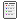###Author Topic: Salary,Wages,Festival Bonus and Annual Leave payment & Over Time Calculation  (Read 1853 times)

#### arif

• Guest##### Salary,Wages,Festival Bonus and Annual Leave payment & Over Time Calculation
« on: April 18, 2017, 10:31:50 PM »
Process on Salary, Wages, Festival Bonus and Annual Leave payment & Over Time Calculation ( Worker )

Salary Calculation: Garments                   Textile

Gross Salary                            =5300.            Gross Salary                      =3505

Basic (Gross-(M+F+C)/1.4)   =3000.             Basic (Gross-(M+C)/1.35) =2300

Medical (Fixed) (M)                  =250.               Medical (Fixed) (M)            =300

Food (Fixed) (F)                       =650.              Convince (Fixed) (C)          =100

Convince (Fixed) (C)                = 200.             House Rent (35% of Basic)=805

House Rent (40% of Basic)     =1200.

Basic/Days of month*Payable Days + Medical+ Food+ Convince+ House Rent =Pay Salary.

Pay Salary + OT Amount + Attendance Bonus                   =Gross Pay.

Gross pay – (Advance Amount + Stamp)                            =Total Pay.

Over Time Calculation:

Basic/208*2                                                                               =OT Rate.

OT Rate*OT Hour                                                                     =OT Amount.

Working & Pay Days:

Actual Day (Without Holiday)                                                    =Monthly Working days.

Actual Duty Days (Without Holiday/Off Day/Leave)                =Present days.

Monthly Working days-Payable days                                        =Absent days.

Present days + (Holiday/Off day/Leave)                                   =Actual Payable days.

Deduction process for unauthorized absent days:

For probationary Employee:

Deduction from Gross Salary

Gross Salary/30*total absent day                                              =Absent amount.

Or Basic Salary/30*total absent day

For permanent Employee:

Deduction from basic salary

Basic salary/30*total absent day                                                =Absent amount.

Earn (Annual) Leave Payment:

Present days/18-Availed AL                                                           =Payable days.

Gross Salary/30*payable days                                                        =Payable amount.

Festival (EID) Bonus Payment:

After Complementation 12 month payable amount is 100% of Basic Salary.